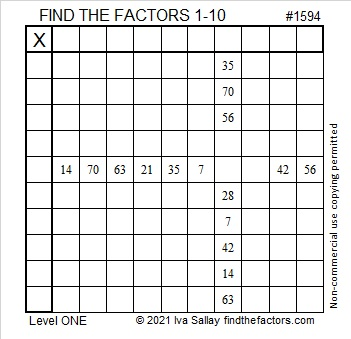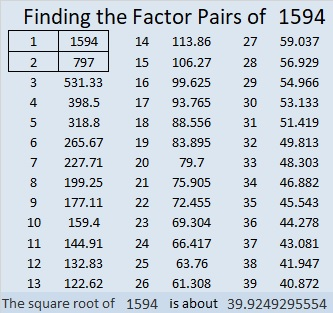# 1594 and Level 1

Contents

### Today’s Puzzle:

Write the numbers from 1 to 10 in the first column and the top row so that those numbers and the given clues function like a multiplication table.### Factors of 1594:

• 1594 is a composite number.
• Prime factorization: 1594 = 2 × 797.
• 1594 has no exponents greater than 1 in its prime factorization, so √1594 cannot be simplified.
• The exponents in the prime factorization are 1 and 1. Adding one to each exponent and multiplying we get (1 + 1)(1 + 1) = 2 × 2 = 4. Therefore 1594 has exactly 4 factors.
• The factors of 1594 are outlined with their factor pair partners in the graphic below.### More about the Number 1594:

1594 is the sum of two squares as well as the double of two squares:
37² + 15² = 1594.
2(26² + 11²) = 1594.

1594 is the hypotenuse of a Pythagorean triple:
1110-1144-1594, calculated from 2(37)(15), 37² – 15², 37² + 15².
It is also 2 times (555-572-797).

This site uses Akismet to reduce spam. Learn how your comment data is processed.